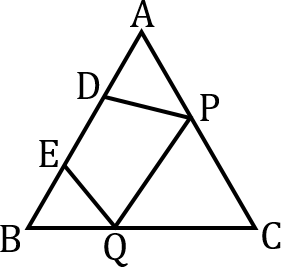Let ABC be a triangle and D and E be two points on side AB such that AD = BC If DP || BC and EQ ||AC, prove PQ || AB.

# Let ABC be a triangle and D and E be two points on side AB such that AD = BC If DP || BC and EQ ||AC, prove PQ || AB.

1. A
2. B
PQ || BC
3. C
PQ || AC
4. D
PQ || AB

Fill Out the Form for Expert Academic Guidance!l

+91

Live ClassesBooksTest SeriesSelf Learning

Verify OTP Code (required)

### Solution:Given that DP || BC and EQ || AC
So,  DB PC
Again EQ || AC
BE BQ
Therefore

EA QC

… (2)
Now AD = BE given AD + DE = BE + DE EA + BD
So DB EA DC QC

## Related content

 Area of Square Area of Isosceles Triangle Pythagoras Theorem Triangle Formula Perimeter of Triangle Formula Area Formulae Volume of Cone Formula Matrices and Determinants_mathematics Critical Points Solved Examples Type of relations_mathematics+91

Live ClassesBooksTest SeriesSelf Learning

Verify OTP Code (required)